# A short introduction to the spathial Package

The spathial package is a generic tool for manifold analysis. It allows the user to infer a relevant transition or evolutionary path which can highlight the features involved in a specific process. spathial can be useful in all scenarios where temporal (or pseudo-temporal) evolution is the main problem (e.g. tumor progression).

The spathial package implements the principal path algorithm  and allows the user to navigate an n-dimensional space. The input for the algorithm is two points, which represent the boundary conditions of the algorithm: the start point and the end point. Given the boundaries, the algorithm learns a smooth transition path connecting them. Along the path, there are new intermediate data samples which gradually morph from the start point to the end point. In this way, it is possible to move from two known states. Additionally, by analyzing the correlation between the path progression and the features, it is possible to perform feature selection. The workflow for constructing the path involves the following steps. Firstly, it is necessary to choose the start point and the end point. spathial provides three different alternatives to do that (which will be discussed later), but the user can additionally choose their own strategy. The second step involves prefiltering the data. This step is not strictly necessary for constructing the path, but it allows the user to obtain a local solution removing some of the data points. In this way, the solution is based on a restricted number of samples. Finally, it is possible to run the principal path algorithm.

spathial additionally provides functions to analyze the path. In particular, it allows the user to plot data in 2d using the t-SNE algorithm  for dimensionality reduction, to learn the labels corresponding to each path’s intermediate points using the kNN algorithm , and to compute the Pearson’s correlation (and the associated p_value and q_value) of the features with respect to the path progression. The latter allows the user to find the features involved in the transition process from the start point to the end point.

The following sections describe how to use spathial and provide some examples.

# Quick start

In this section, the most basic steps to run the spathial implementation of the principal path algorithm  are demonstrated with a simple 2d example.

The very first step is the spathial package installation.

• Directly from CRAN:
``install.packages("spathial")``
• From GitHub using devtools:
``````install.packages("devtools")
library(devtools)
devtools::install_github("erikagardini/spathial", build_vignettes=TRUE)``````

``library("spathial")``

To compute the principal path, one assumes an input matrix `X`. Each column of the matrix `X` is a feature (e.g. a gene) and each row has a univocal name (e.g. a sample). For a supervised solution, one also assumes that the input vector `X_labels` is present, containing, for each row of `X`, a description label (e.g. the sample category). Otherwise, the unsupervised solution can be obtained with `X_labels = NULL`. For simplicity, a simple .csv file with 900 samples and 3 columns (2 + labels) is provided. The following code snippet shows how to load the .csv and how to properly format the data.

``````# Load the dataset with 900 samples
myfile<-system.file("extdata", "2D_constellation.csv", package = "spathial")
``````##    feature1  feature2 label
## 1  0.440620 -0.206480    c8
## 2 -0.305250  0.122770    c3
## 3 -0.234850  0.097566    c8
## 4  0.205200 -0.058423    c8
## 5  0.022774  0.134450    c8
## 6 -0.223260  0.092053    c8``````
``````# Vector X_labels
X_labels <- data\$label
X_labels_num <- data\$numLabels
# Data Matrix X
X <- data[,2:(ncol(data)-2)]
rownames(X)<-paste0("sam",rownames(X))``````

This is how the data matrix `X` should look at this point

``head(X)``
``````##       feature2  feature1
## sam1 -0.206480  0.440620
## sam2  0.122770 -0.305250
## sam3  0.097566 -0.234850
## sam4 -0.058423  0.205200
## sam5  0.134450  0.022774
## sam6  0.092053 -0.223260``````

The sample labels vector `X_labels`, assigns categories to each sample, coherently with row order in `X`

``head(X_labels)``
``##  "c8" "c3" "c8" "c8" "c8" "c8"``

The following code snippet shows how to plot the data points colored according to `X_labels`:

``````# Plot the results
colors <- rainbow(length(table(as.numeric(as.factor(X_labels)))))
colors_labels <- sapply(as.numeric(as.factor(X_labels)), function(x){colors[x]})
par(mar=c(5.1, 4.1, 4.1, 8.1), xpd=TRUE)
plot(X[,1],X[,2],col=colors_labels,pch=as.character(as.numeric(as.factor(X_labels))),
xlab=colnames(X),ylab=colnames(X), main="Data points")
legend_names = unique(X_labels)
legend_color = unique(colors_labels)
legend_pch = unique(as.character(as.numeric(as.factor(X_labels))))
legend("topright", inset=c(-0.25,0), legend=legend_names, col=legend_color, pch=legend_pch)``````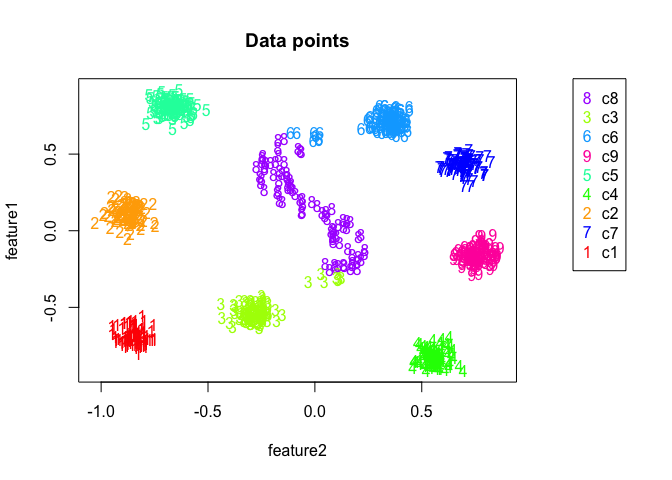Quick Start example dataset 2D. Each point is a sample of the dataset, colored according to its labels.

``par(oldpar)``

## Step 1: select the boundaries

The first step in computing the principal path is to select the start point and the end point. The package spathial has the function `spathialBoundaryIds` which takes as input the matrix `X`, the corresponding labels `X_labels` (or NULL), and the parameters `mode`, `from` and `to`.

The parameter `mode` can assume one of the following values:

• 1 (default): the user can choose the start and end points directly from the 2D representation of the data points. In this case, the values of the parameters `from` and `to` are not considered.
• 2: the start point is the centroid of all the data points labelled as the parameter `from`, while the end point is the centroid of all the data points labelled as the parameter `to`. This mode is allowed only when `X_labels` is not NULL.
• 3: the start point is the data point with the univocal rowname equal to the parameter `from` while the end point is the data point with the univocal rowname equal to the parameter `to`.

The output of the function `spathialBoundaryIds` is a list with the following content:

• X: the initial input matrix `X` plus the start and end points;
• X_labels: the initial vector `X_labels` inclusive of the labels of the start and end points;
• boundary_ids: the rowname of the start and end points.

N.B. The matrix `X` and the vector `X_labels` change only when `mode=2` (the resulting matrix and vector have two additional elements, corresponding to the centroids).

The user can choose the start and end points with their own strategy, which can be more suitable for their specific problem; in this case, the user should run the function `spathialBoundaryIds` with `mode=3` specifing the rownames of the samples using the parameters `from` and `to`.

The following code chunks show how to use the function `spathialBoundaryIds`, with different values of the parameter `mode`, and how to extract the output:

``````# mode=1 (User-selected)
boundary_init <- spathial::spathialBoundaryIds(X, X_labels, mode=1)``````
``````# mode=2 (From named label centroid to another label centroid)
boundary_init <- spathial::spathialBoundaryIds(X, X_labels, mode=2, from="c3", to="c6")``````
``````# mode=3 (From named sample to another named sample)
boundary_init <- spathial::spathialBoundaryIds(X, X_labels, mode=3,
from="sample123", to="sample456")``````

Once the boundaries are defined, and only in the case of `mode=2`, the `X` and `X_labels` objects inside the `boundary_init` object contain extra metasamples (the boundaries) and thus the original `X` and `X_labels` need to be updated accordingly:

``````# Take the output from the variable boundary_init
boundary_ids<-boundary_init\$boundary_ids
X<-boundary_init\$X
X_labels<-boundary_init\$X_labels``````

The following plots the boundaries when `mode=2`, `from=3`, and `to=6`:

``````#Plot the results
boundaries <- X[which(rownames(X) == boundary_ids | rownames(X) == boundary_ids),]
par(mar=c(5.1, 4.1, 4.1, 8.1), xpd=TRUE)
plot(X[,1],X[,2], col=colors_labels, pch=as.character(as.numeric(as.factor(X_labels))), xlab=colnames(X), ylab=colnames(X), main="Boundary points")
points(boundaries[,1],boundaries[,2], pch="x",col="black",cex=4)
legend_names = c(unique(X_labels), "boundaries")
legend_color = c(unique(colors_labels), "black")
legend_pch = c(unique(as.character(as.numeric(as.factor(X_labels)))), "X")
legend("topright", inset=c(-0.3,0), legend=legend_names, col=legend_color, pch=legend_pch)``````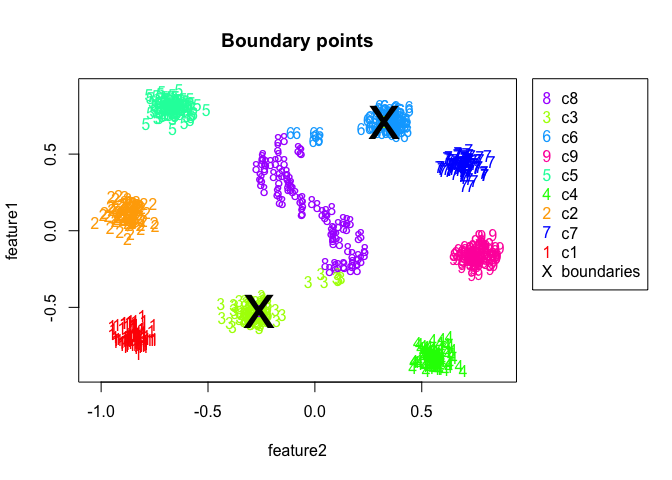Quick Start example - boundaries. Each point is a sample of the 2D dataset, colored according to its labels. Boundaries are respectively the centroid of the samples labelled as 3 (start point) and the centroid of the samples labelled as 6 (end point).

``par(oldpar)``

## Step 3: prefiltering (optional)

The principal path algorithm is intrinsically global. If one is searching for a path which does not involve the whole dataset, one can run the function `spathialPrefiltering` before the principal path algorithm is applied. If one wants to run spathial on the entire dataset, this filtering procedure is not due.

The function `spathialPrefiltering` takes as input the matrix `X` and the boundaries `boundary_ids` which are the result of the function `spathialBoundaryIds`.

The output of the function `spathialPrefiltering` is a list with the following content:

• mask: the indexes of the samples to preserve;
• boundary_ids_filtered: the rowname of the start and end points.

The following code shows how to use the `spathialPrefiltering` function and how to extract the output. The function remove some samples and they are stored in the `X_garbage` matrix. The `mask` vector contains the samples to be kept.

``````# Prefilter data
filter_res <- spathial::spathialPrefiltering(X, boundary_ids)
boundary_ids <- filter_res\$boundary_ids

# Plot the results
boundaries <- X[which(rownames(X) == boundary_ids | rownames(X) == boundary_ids),]

The following code shows the results of the prefiltering step, with removed points in gray:

``````oldpar <- par(no.readonly = TRUE)
par(mfrow=c(2,1))
par(mar=c(5.1, 4.1, 4.1, 8.1), xpd=TRUE)
plot(X[,1],X[,2], col=colors_labels, pch=as.character(as.numeric(as.factor(X_labels))), xlab=colnames(X), ylab=colnames(X), main="Before Filtering")
points(boundaries[,1],boundaries[,2], pch="x",col="black",cex=4)
legend_names = c(unique(X_labels), "boundaries")
legend_color = c(unique(colors_labels), "black")
legend_pch = c(unique(as.character(as.numeric(as.factor(X_labels)))), "X")
legend("topright", inset=c(-0.3,0), legend=legend_names, col=legend_color, pch=legend_pch)

par(mar=c(5.1, 4.1, 4.1, 8.1), xpd=TRUE)
plot(X[,1],X[,2], col=colors_labels, pch=as.character(as.numeric(as.factor(X_labels))), main="After Filtering", xlab=colnames(X),ylab=colnames(X))
points(boundaries[,1],boundaries[,2], pch="x",col="black",cex=4)
points(X_garbage[,1],X_garbage[,2], col="gray", pch=4)
legend_names = c(unique(X_labels), "boundaries", "filtered")
legend_color = c(unique(colors_labels), "black", "gray")
legend_pch = c(unique(as.character(as.numeric(as.factor(X_labels)))), "X", "x")
legend("topright", inset=c(-0.3,0), legend=legend_names, col=legend_color, pch=legend_pch)``````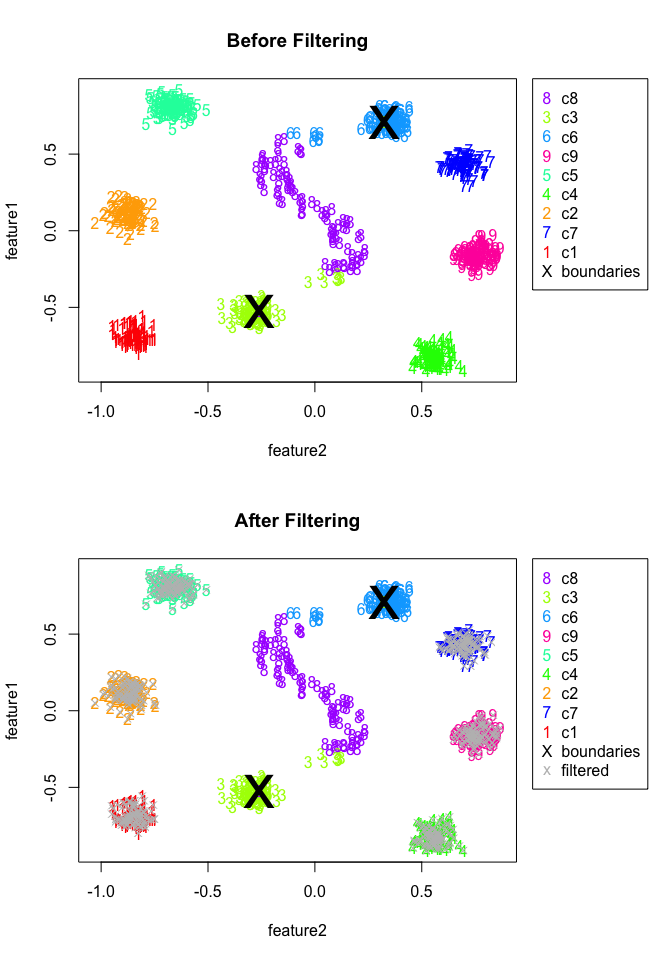Quick Start example - prefiltering. Each point is a sample of the 2D dataset, colored according to its labels. Boundaries are respectively the centroid of the samples labelled as 3 (start point) and the centroid of the samples labelled as 6 (end point). Prefiltered samples are marked in gray.

``par(oldpar)``

## Step 4: principal path

The function `spathialWay` executes the principal path algorithm and gives as output the waypoints. It takes as input the matrix `X`, the boundaries `boundary_ids` and the parameter `NC`, which is the desired number of waypoints of the resulting principal path. For example, given `NC=10` the resulting path will be composed of 10 waypoints plus the starting and the ending points.

The output of the function `spathialWay` is the variable `spathial_res`, which contains the coordinates of the waypoints of the principal path;

The following code shows how to use the function `spathialWay`, with or without prefiltering, and how to get the output:

``````# Compute principal path without prefiltering
NC <- 50
spathial_res_without_filtering <- spathial::spathialWay(X, boundary_ids, NC)``````
``````# Compute principal path after prefiltering
NC <- 50
spathial_res_with_filtering <- spathial::spathialWay(X_filtered, boundary_ids, NC)``````

The next subsection shows how to get results from the output object `spathial_res`.

## Step 5: understanding the results

The package spathial includes three different functions to interpret the output of the principal path algorithm: `spathialPlot`, `spathialLables`, and `spathialStatistics`. The following subsections describe what they do and how to use each of them.

### Plotting the principal path

The function `spathialPlot` plots the principal path together with all the data points (either filtered or not filtered) together with the boundaries. This function takes as input the matrix `X` (the initial version), the vector `X_labels` (or NULL), the boundaries `boundary_ids`, the output of the principal path algorithm `spathial_res`, the parameter `perplexity value` (default 30), the parameter `mask`, which is one of the results of the prefiltering and is `NULL` when the prefiltering is not computed, and the parameter `title`, which is the title of the plot and can be `NULL`. When the input matrix `X` has more than 2 columns, the function reduces the dimension of the space from N (>2) to 2 using the t-SNE algorithm .

The following code shows how to use the `spathialPlot` function with the path generated in the previous step (with or without prefiltering):

``````# Plot principal path with prefiltering - provide a mask
spathial::spathialPlot(X, X_labels, boundary_ids, spathial_res_with_filtering,
title="Principal path with prefiltering"
)``````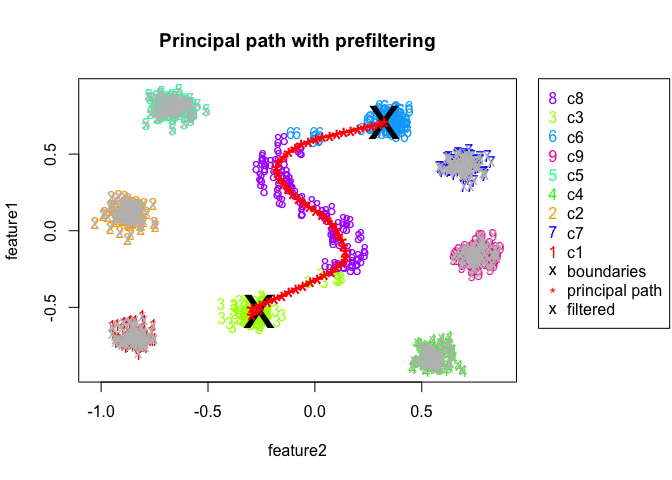Output of the spathialPlot() function. Each point is a sample of the 2D dataset, colored according to its labels. Boundaries are respectively the centroid of the samples labelled as 3 (start point) and the centroid of the samples labelled as 6 (end point). The Principal Path is marked in blue. It moves from the start point to the end point passing through each waypoint (marked with “*“). Filtered samples are marked in grey.

``````# Plot principal path without prefiltering - mask NULL
spathial::spathialPlot(X, X_labels, boundary_ids, spathial_res_without_filtering,
perplexity_value=30,
title="Principal path without prefiltering"
)``````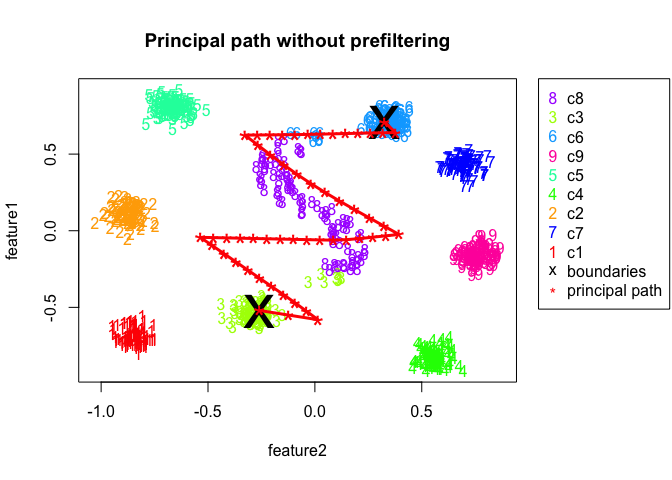Output of the spathialPlot() function. Each point is a sample of the 2D dataset, colored according to its labels. Boundaries are respectively the centroid of the samples labelled as 3 (start point) and the centroid of the samples labelled as 6 (end point). The Principal Path is marked in blue. It moves from the start point to the end point passing through each waypoint (marked with “*“). Filtered samples are marked in grey.

### Assessing the path progression

The function `spathialLabels` predicts the label for each waypoint of the principal path by detecting the nearest sample . This function is available only when `X_labels != NULL` and could be particularly useful when one wants to find the breakpoints between one class and the other. The function takes as input the matrix `X` (which comprises only the preserved samples if the prefiltering was used), the vector `X_labels`, and the output object of the principal path algorithm `spathial_res`. The output are the labels for each waypoint of the principal path.

The following code shows how to use the `spathialLabels` function and how to plot the result:

``````oldpar <- par(no.readonly = TRUE)
par(mfrow=c(2,1))
# Matrix X not filtered
ppath_labels <- spathial::spathialLabels(X, X_labels, spathial_res_with_filtering)
# Matrix X filtered
ppath_labels_filtered <- spathial::spathialLabels(X, X_labels, spathial_res_without_filtering)

# Plot the results
ppath_labels_numerical = as.numeric(as.factor(ppath_labels))
colors_labels_ppath <- sapply(ppath_labels_numerical, function(y){colors[as.integer(y)]})
plot(c(1:length(ppath_labels_numerical)), c(ppath_labels_numerical), col=colors_labels_ppath,
pch=as.character(ppath_labels_numerical), xlab="Path Step", ylab="Sample Label",
main="Path progression with prefiltering"
)

# Plot the results
ppath_labels_filtered_numerical = as.numeric(as.factor(ppath_labels_filtered))
colors_labels_ppath <- sapply(ppath_labels_filtered_numerical, function(y){colors[as.integer(y)]})
plot(c(1:length(ppath_labels_filtered_numerical)), c(ppath_labels_filtered_numerical), col=colors_labels_ppath,
pch=as.character(ppath_labels_filtered_numerical), xlab="Path Step", ylab="Sample Label",
main="Path progression without prefiltering"
)``````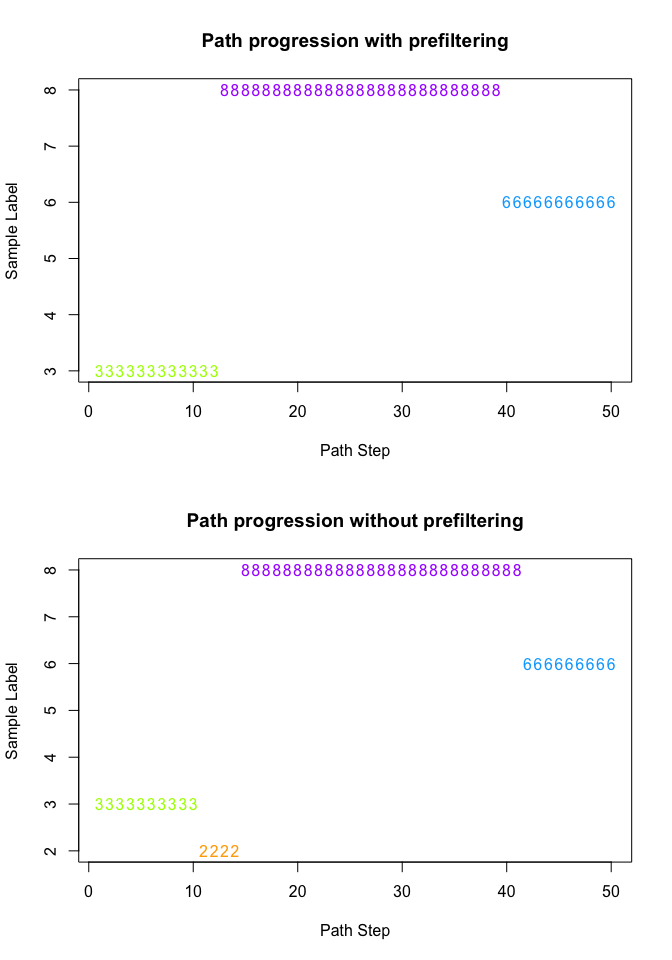Quick Start example - path labels across path steps. For each waypoint of the Principal Path (computed from the centroid of the samples labelled as 3 (start point) to the centroid of the samples labelled as 6 (end point), the assigned label is the label of the nearest sample.

``par(oldpar)``

### Extract principal-path-associated features

The function `spathialStatistics` returns statistics for each feature, based on their relation with the principal path calculated and stored in `spathial_res`. In particular, here one wants to understand how much each feature (a coordinate of the N-dimensional space) is correlated with the evolution of the principal path, that is, to detect evolutive features. This is a path-centric way to perform feature selection.

For this reason, the output of the function `spathialStatistics` is a list that attaches a progression score to features.

• correlations: This vector contains the Pearson’s correlation coefficients between each feature and the path.
• rank_scores: This vector contains the ranks of associations between the n features and the path (with 1 being the most positively correlated, and n the most negatively correlated).
• p_value: This vector contains the p values from the Pearson’s correlation scores.
• p_adj: This vector containes the p values adjusted according to the Benjamini & Hochberg (BH) method.

The following code shows how to use the function `spathialStatistics` and to extract the correlation-based association between features and the path.

``````# Calculate Association Statistics for each feature in the path
statistics <- spathial::spathialStatistics(spathial_res_with_filtering)

# Extract Pearson correlation coefficients between features and path
statistics\$correlations``````
``````##  feature2  feature1
## 0.5134595 0.9944206``````
``````# Calculate Association Statistics for each feature in the path
statistics <- spathial::spathialStatistics(spathial_res_without_filtering)

# Extract Pearson correlation coefficients between features and path
statistics\$correlations``````
``````##  feature2  feature1
## 0.5062762 0.9672803``````

# A slightly more complex example

In this section, a higher-dimensional example with 100 features is shown. In this case, the input matrix `X` is a reduced version of the TCGA Liver Cancer RNA-Seq dataset, which comprises only the 100 features (gene expression profiles, RPM-normalized) with the highest variance across the dataset. The vector `X_labels` contains the information about the samples. In particular, the label is “Tumor” for tumor samples, and “Normal” for normal samples, collected in the same dataset.

In this case, the aim of the example is to navigate the space from the centroid of the normal tissue samples (label “Normal”) to the centroid of the tumor samples (label “Tumor”) in order to gradually morph from one histological state to the other. For this reason, one can use the function `spathialBoundaries` with `mode=2` specifying `from="Normal"` and `to="Tumor"`.

The prefiltering will not be executed since one is searching for a global solution.

The following code block shows how to compute the principal path algorithm. First, one loads the data from a .csv file containing RPM-normalized gene expression data:

``````# Load data
myfile<-system.file("extdata", "liver_tcga_example1.csv", package = "spathial")
data[1:4,1:5]``````
``````##                                   ALB       HP   MT.ATP6     APOA2  MT.ATP8
## TCGA-UB-A7MB-01A-11R-A33R-07 593956.4  92680.9  170568.5  691801.2 221968.2
## TCGA-CC-A9FW-01A-11R-A37K-07 513497.3 280958.0  301123.4  598087.7 318292.9
## TCGA-DD-AAEE-01A-11R-A41C-07 456418.2 200048.9  422911.6 1187913.9 334117.4
## TCGA-FV-A3R3-01A-11R-A22L-07 184082.4 172949.5 1041332.0 1116072.0 757033.8``````

Then, one transforms it into two objects: the numeric gene expression profiles (`X`) and the associations between the samples and the sample category (`X_labels`), where “Tumor” indicates a tumor sample, and “Normal” a normal tissue sample.

``````X <- data[,1:(ncol(data)-1)]
X_labels <- data[,"Category"]
X[1:4,1:5]``````
``````##                                   ALB       HP   MT.ATP6     APOA2  MT.ATP8
## TCGA-UB-A7MB-01A-11R-A33R-07 593956.4  92680.9  170568.5  691801.2 221968.2
## TCGA-CC-A9FW-01A-11R-A37K-07 513497.3 280958.0  301123.4  598087.7 318292.9
## TCGA-DD-AAEE-01A-11R-A41C-07 456418.2 200048.9  422911.6 1187913.9 334117.4
## TCGA-FV-A3R3-01A-11R-A22L-07 184082.4 172949.5 1041332.0 1116072.0 757033.8``````
``````# Choose the starting and ending points
boundary_init <- spathial::spathialBoundaryIds(X, X_labels, mode=2, from=2, to=1)
# Alternative, mode 3:
# from="TCGA-DD-A39W-11A-11R-A213-07", to="TCGA-G3-AAV2-01A-11R-A37K-07"
boundary_ids <- boundary_init\$boundary_ids
X <- boundary_init\$X
X_labels <- boundary_init\$X_labels

# run spathial
NC <- 50
spathial_res <- spathial::spathialWay(X, boundary_ids, NC)``````

In order to visualize the multidimensional dataset in two dimensions, one performs a t-SNE projection on it .

``````# Plot the path in 2D using Rtsne
spathial::spathialPlot(X, X_labels, boundary_ids, spathial_res, perplexity_value=30)``````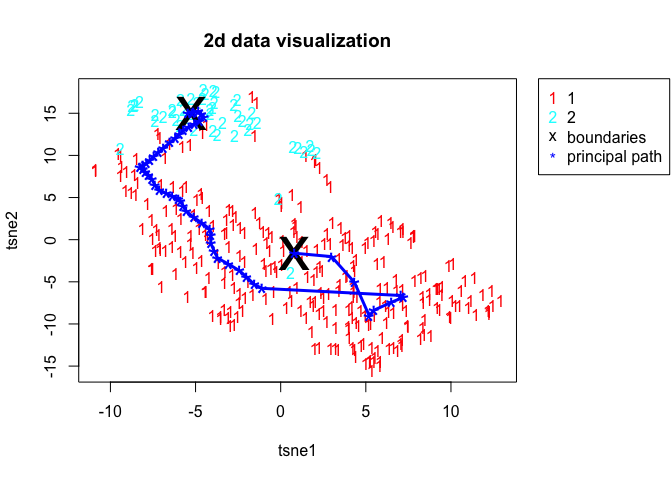Principal Path across the TCGA Liver Cancer dataset. 2D visualization of the Principal Path together with the data points. The x and y coordinates are the output of the dimensionality reduction performed with tSNE . The start point and the end point of the Principal Path are the centroid of the normal samples and the centroid of the tumor samples, respectively. The Principal Path comprises 50 intermediate points (waypoints) plus the boundaries.

``````# Labels for each waypoint with knn
ppath_labels <- spathial::spathialLabels(X, X_labels, spathial_res)
# Plot the results
colors <- rainbow(length(table(as.numeric(as.factor(X_labels)))))
ppath_labels <- as.vector(ppath_labels)
colors_labels_ppath <- sapply(ppath_labels, function(y){colors[as.integer(y)]})
plot(c(1:length(ppath_labels)), c(ppath_labels), col=colors_labels_ppath,
pch=as.character(ppath_labels), xlab="Path Step", ylab="Sample Label",
main="Path progression"
)``````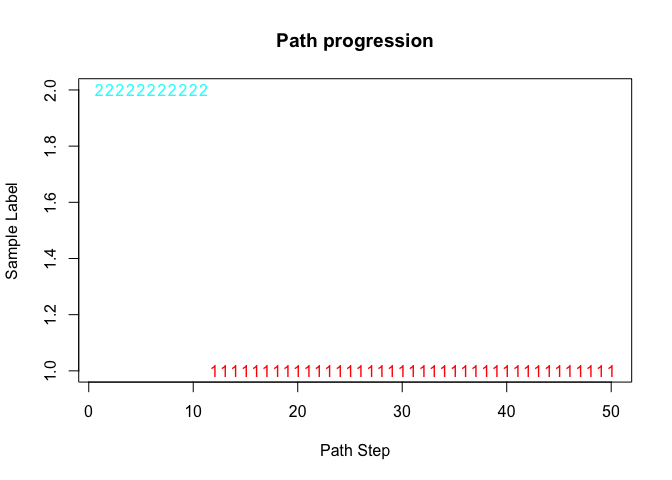Liver Cancer example - path labels across path steps. For each waypoint of the Principal Path (computed from the centroid of the normal samples to the centroid of the tumor samples), the assigned label is the label of the nearest sample.

Then, one can quickly extract the gene expression profiles most correlated with the path:

``````# Correlation along the path
statistics<-spathialStatistics(spathial_res)``````

In this case, the statistics is particularly interesting, as it shows the functional genes most associated with the transition between normal and tumor liver tissues. In fact, it contains information about the correlation between each feature (genes) and a vector from 1 to NC+2 (where NC is the number of waypoints), which represents the progression along the path. This helps find the genes that are particularly involved in the evolution from the healthy to the unhealthy state. The following code highlights the genes most positively and most negatively associated with the normal-tumor path progression (10 genes each):

``````singlepath_correlations<-statistics\$correlations
top_positive_correlations<-sort(singlepath_correlations,decreasing=TRUE)[1:10]
top_positive_correlations``````
``````##     RPS19     RPL30     RPS18    RPL13A     RPS27      RPL8     RPL39     RPL10
## 0.9489431 0.9334733 0.9334049 0.9213973 0.9158444 0.9149281 0.9145781 0.9133683
##     RPS16     RPS21
## 0.9061229 0.9025649``````
``````top_negative_correlations<-sort(singlepath_correlations,decreasing=FALSE)[1:10]
top_negative_correlations``````
``````##       RBP4      ALDOB      APOC3      ADH1B        FGA         HP        FGG
## -0.9851525 -0.9655488 -0.9615431 -0.9576245 -0.9492497 -0.9374311 -0.9301608
##        TTR        FGB       MT2A
## -0.9298318 -0.9235418 -0.9208846``````

# A real case study

This section shows a real case study on the TCGA Lung Cancer RNA-Seq dataset. Here, the input matrix `X` is the fully TCGA Lung Cancer RNA-Seq dataset, with 19637 features (gene expression profiles, RPM-normalized) and 562 samples. Each column of matrix `X` represents a feature, and each row is the gene expression profile of a specific sample. The vector `X_labels` contains the annotations of the samples (“Tumor” or “Normal”) that can be extracted from the TCGA barcode. We provide an `.rda` file with both the `X` and `X_labels`. One can simply load it by computing the following command.

``load(url("https://github.com/erikagardini/spathial_dataframes/raw/master/luad_tcga.rda"))``

The aim of the example is to navigate the space from the normal samples to the tumor samples.

Here, the start point is the most distant normal sample from the tumor centroid and the end point is the most distant tumor sample from the normal centroid. These start and end points are selected because one is searching for the extremes, conceptually the most normal sample and the most not-normal sample.

Since this is not an existing `spathialBoundaries` mode, one can extract the rownames of the start and end points, then one can use the function `spathialBoundaries` with `mode=3` and setting `from` and `to`, respectively equal to the rownames of the start and end points.

The following code block shows how to find the rowname of our start and end points:

``````#Compute centroids
normal_centroid <- colMeans(X[which(X_labels == "normal"),], na.rm = TRUE)
tumor_centroid <- colMeans(X[which(X_labels == "tumor"),], na.rm = TRUE)

#Subdivide normal samples from tumor samples
normal <- X[which(X_labels == "normal"),]
tumor <- X[which(X_labels == "tumor"),]

#Get start and end point names
getMaxDistancePoint <- function(centroid, samples){
library(pracma)

centroid <- t(centroid)
dst<-pracma::distmat(
as.matrix(centroid),
as.matrix(samples)
)
ord <- order(-dst)
return(rownames(samples)[ord])
}

startPoint <- getMaxDistancePoint(tumor_centroid, normal)
endPoint <- getMaxDistancePoint(normal_centroid, tumor)``````

Now, one can use the spathial method `spathialBoundaryIds` with `mode=3` to initialize the boudaries as shown below:

``````boundaries <- spathial::spathialBoundaryIds(X, X_labels, mode=3, from=startPoint, to=endPoint)
boundary_ids<-boundaries\$boundary_ids
X <- boundaries\$X
X_labels <- boundaries\$X_labels``````

In this case, the prefiltering will not be executed because one is searching for a global solution. The next step is to run the principal path algorithm using the `spathial` method `spathialWay`. A path with 50 intermediate points (waypoints) can be obtained with `NC=50`.

``````spathial_res <- spathial::spathialWay(X, boundary_ids, NC = 50)
save(spathial_res, "spathial_res.rda")``````

Each waypoint is a new gene profile that is slightly different from the previous one, and all of them are topologically connected via a chain of springs.

The spathial method `spathialPlot` can be used to visualize the path.

``````load(url("https://github.com/erikagardini/spathial_dataframes/raw/master/spathial_res.rda"))
spathial::spathialPlot(X, X_labels, boundary_ids, spathial_res, perplexity_value = 30, mask = NULL)``````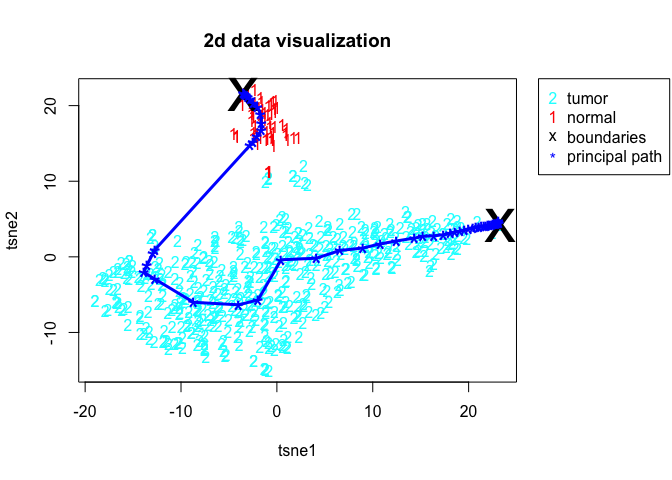Principal Path across the TCGA Luad Cancer dataset. 2D visualization of the Principal Path together with the data points. The x and y coordinates are the output of the dimensionality reduction performed with tSNE . The start and end points of the Principal Path are the most distant points from the centroid of the tumor samples and the centroid of the normal samples, respectively. The Principal Path comprises 50 intermediate points (waypoints) plus the boundaries.

Since we have an n-dimensional space (with n = 19637) in this example, a dimensionality reduction strategy is necessary to plot the principal path algorithm. spathial uses the t-SNE algorithm .

The spathial method `spathialStatistics` can be used to calculate the Pearson’s correlation coefficients, the p values, and the adjusted p values and to rank the genes according to the correlation scores:

``spathial_statistics <- spathial::spathialStatistics(spathial_res)``

In this way, it is possible to find the genes involved in the transition from “Normal” to “Tumor” (genes with high correlation coeffients and significant p values).

# An example with Single-cell RNA-Seq data

In this section, a real case study is shown on the Karlsson Single-cell RNA-Seq dataset . Here, the input matrix `X` comprises 23928 features (genes) and 96 samples. Each column of matrix `X` represents a feature, and each row is the gene expression profile of a specific cell. The vector `X_labels` contains the annotations of the cell (“G1”, “S” or “G2/M”), which describes the phase of the cell cycle. We provide an `.rda` file with both the `X` and `X_labels`. Users can simply load it by computing the following commands.

``````load(url("https://github.com/erikagardini/spathial_dataframes/raw/master/karlsson-rawcounts.rda"))

#NORMALIZATION AND PREFILTERING OF THE DATA (GENES IN AT LEAST 3 CELLS)
#library(Seurat)
#seuset<-CreateSeuratObject(counts=rawcounts,min.cells=3,min.features=200)
#normalized_seuset<-NormalizeData(seuset,normalization.method="LogNormalize",scale.factor=10000)
#normalized_expmat <- as.matrix(normalized_seuset[["RNA"]]@data)
#save(normalized_expmat,annotation,file="karlsson-normalized_expmat.rda")

X <- t(normalized_expmat)
X_labels <- annotation``````

The aim of the example is to navigate the space from the “G1” phase to the “G2/M” phase in order to find the genes involved in the cell cycle.

Here, the start point is the centroid of the “G1” cells and the end point is the centroid of the “G2” cells. This is an existing `spathialBoundaries` mode, therefore the function `spathialBoundaries` can be used with `mode=2` and the parameters `from` and `to` equal to “G1” and “G2”, respectively.

The following code block shows how to set the boundaries:

``````boundaries <- spathial::spathialBoundaryIds(X, X_labels, mode=2, from="G1", to="G2/M")
boundary_ids<-boundaries\$boundary_ids
X <- boundaries\$X
X_labels <- boundaries\$X_labels``````

In this case, the prefiltering will not be executed since one is searching for a global solution. The next step is to run the principal path algorithm using the spathial method `spathialWay`. A path with 50 intermediate points (waypoints) can be obtained with `NC=50`.

``spathial_res <- spathial::spathialWay(X, boundary_ids, NC = 50)``

Each waypoint is a new cell that is slightly different from the previous one, and all of them are topologically connected via a chain of springs.

Finally, one can use the spathial method `spathialPlot` to plot the path as follows:

``spathial::spathialPlot(X, X_labels, boundary_ids, spathial_res, perplexity_value = 30, mask = NULL)``